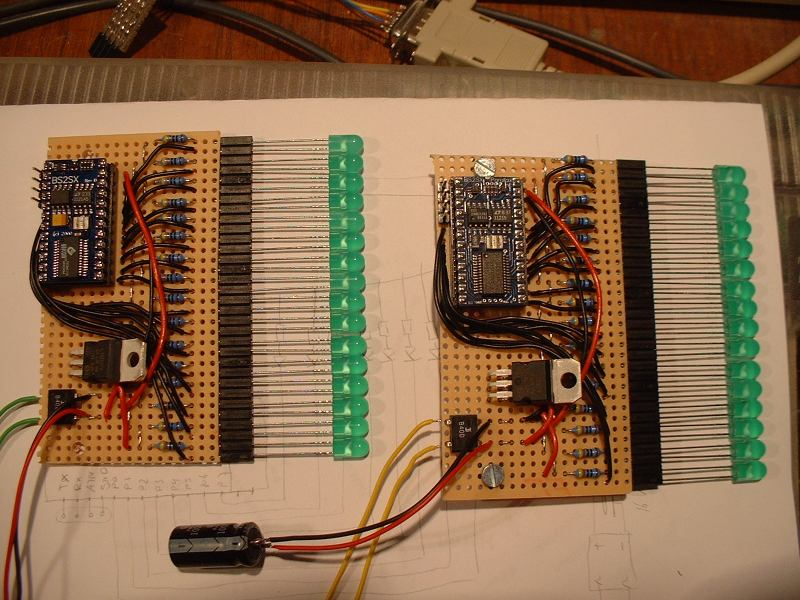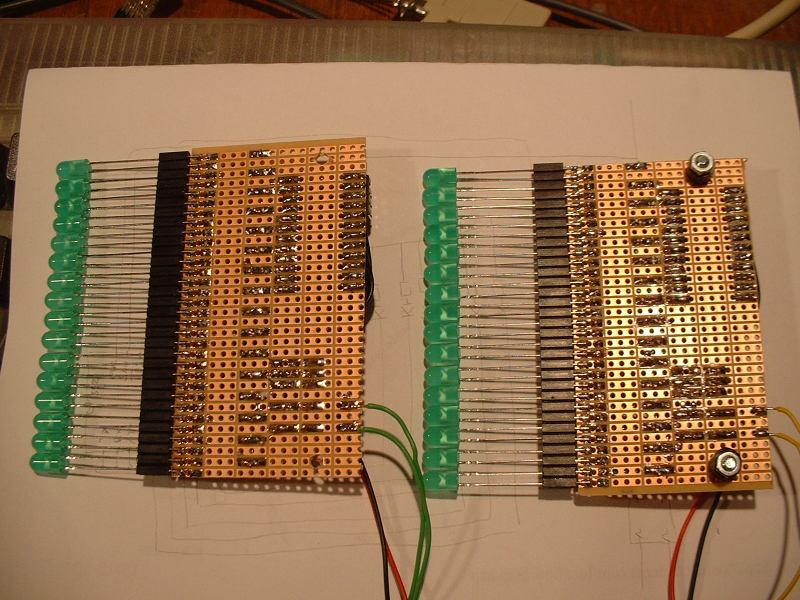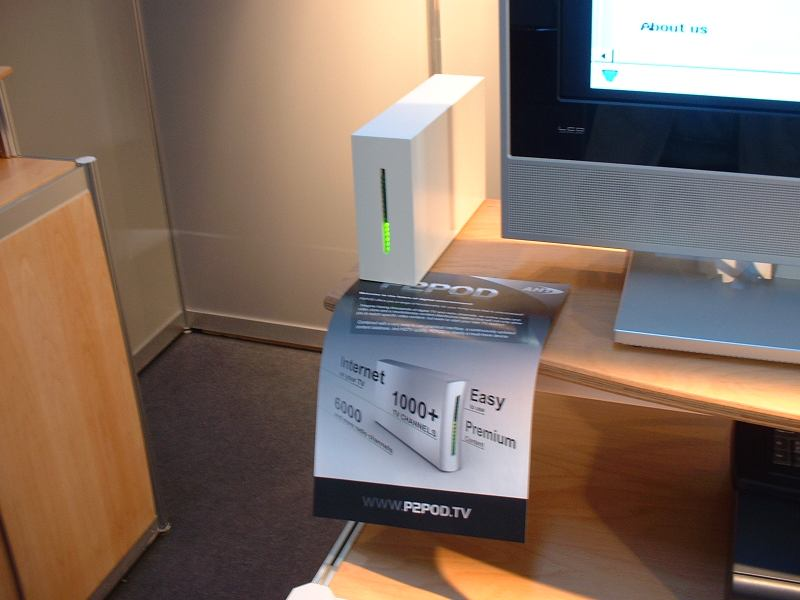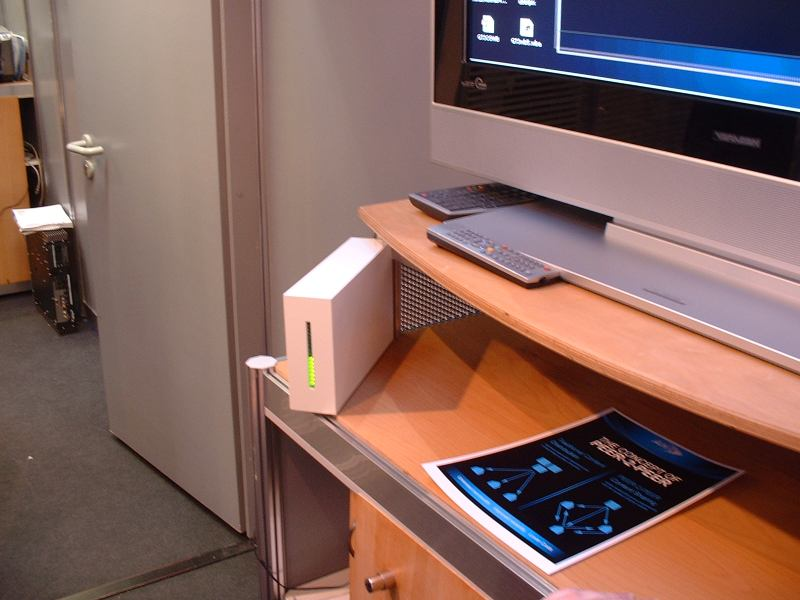28 februari 2006

# P2Pod mockups

On the 2006 Cebit exhibition we wanted to attract attention to our settopboxes, because
the small devices werent ready for the show it was decided that we would have small boxes
with a big LED display as mockups.
Where the leds where supposed to show the load on the net.

There where only a couple of days to get things ready before they had to be shipped
so i decided to use Basic stamp 2 devices, because its really simple to get something working on them fast.
It was decided to use 16 LEDs which have their anodes connected to an external voltage regulator and
their cathodes to the basic stamp. So the current isnt flowing through the regulator on the stamp.

On this picture one device is ready and programmed, while two others are in the process
of being build:Here are two finished boards:And this is the back side.Here are the actual 3 mockups at the 2006 Cebit:This is the actual program that runs on the basic stamps:

' {\$STAMP BS2sx}

t VAR Byte
r VAR Byte
leds VAR Byte

v VAR Word
time VAR Word

DIRS = 65535 ' all 16 lines outputs
OUTS = 0 ' all 16 line on
PAUSE 500 ' half second test
OUTS = 65535 ' all 16 lines off

FOR t = 0 TO 17 STEP 1
leds = t
GOSUB setled
PAUSE 30
NEXT

FOR t = 16 TO 0 STEP -1
leds = t
GOSUB setled
PAUSE 30
NEXT

loop:

leds = 8 + (SIN(time)/64) + (SIN(time/2)/64) + (SIN(time/4)/64) + (SIN(time/8)/64)
GOSUB setleds
'PAUSE 20 '50th sec
time = time + 1

GOTO loop:

setleds:
v = 0
FOR r = 0 TO leds
v = v << 1
v = v | 1 ' OR 1 .. AND 0 ?
NEXT
OUTS = 65535 - v ' invert ..
RETURN

setled:
v = 1
FOR r = 0 TO leds
v = v << 1
NEXT
OUTS = 65535 - v
RETURN